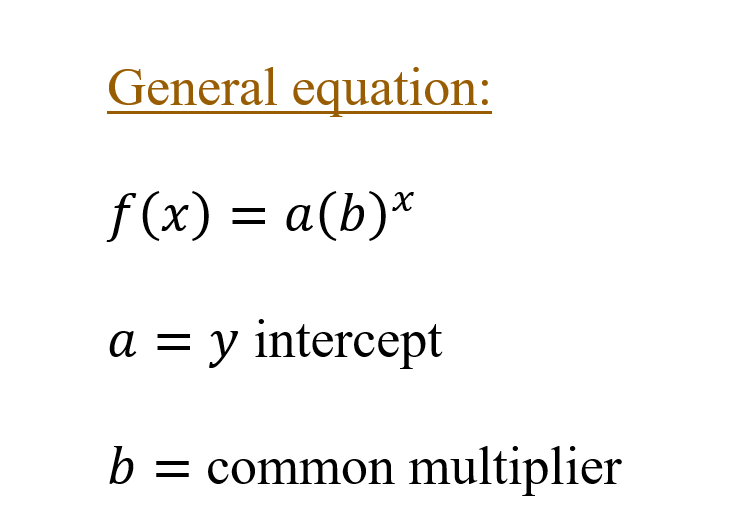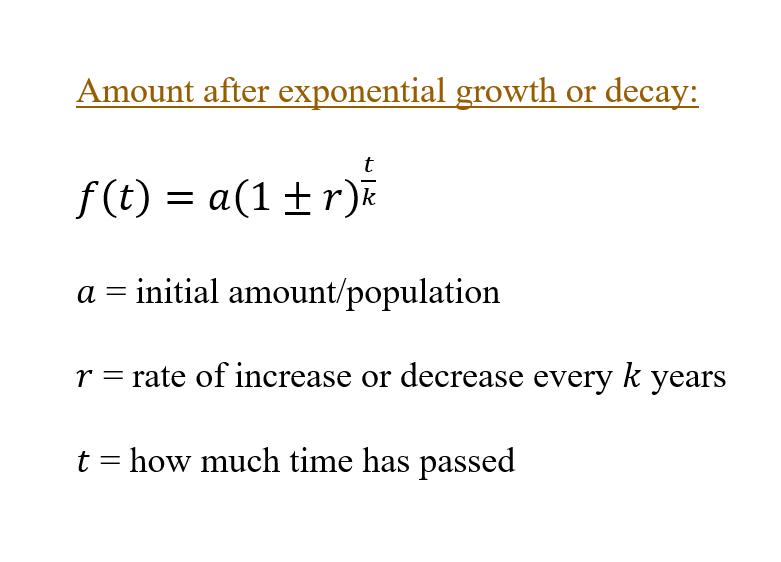Exponential Functions and Equations

What is an Exponential equation?

An exponential equation is an equation in which a variable occurs in the exponent.

What is a exponential function?

An exponential function is a function in which a variable occurs in the exponent.Laws of exponentsThe final value after an exponential growth or decay?Finding the account Balance after a time period, if the account earns an annual interest that is compounded.